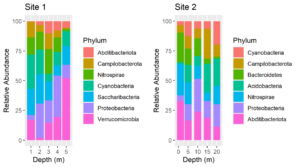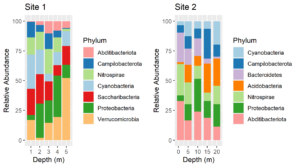# Using Named Colors with ggplot2

Consider these two plots:The colors assigned to the phyla are not the same in the two plots. We cannot make them so simply by faceting because there are unique phyla at each site and the sites were sampled at different depths.  In fact, they were plotted using different tibbles. Still, it would be much easier to interpret the plots if the same colors were assigned to the phyla that are common between the plots. We can do this by using a palette of named colors.

First, get a vector of the union of unique phyla for each plot and determine its length. The plots were made from two tibbles, `df1` and `df2`.

````unique.phyla <- union(unique(df1\$Phylum), unique(df2\$Phylum))````
length(unique.phyla)``````
 9``````

So while there are only 7 phyla in each plot, there are a total of 9 in the two plots. Next, get a vector of 9 colors and name the colors with the names of the phyla.

``````my.colors <- RColorBrewer::brewer.pal(n = length(unique.phyla),name = "Paired")
names(my.colors) <- unique.phyla
my.colors
Cyanobacteria  Campilobacterota  Nitrospirae Proteobacteria
"#A6CEE3"      "#1F78B4"         "#B2DF8A"       "#33A02C"
Abditibacteriota Saccharibacteria Verrucomicrobia Acidobacteria
"#FB9A99"        "#E31A1C"        "#FDBF6F"       "#FF7F00"
Bacteroidetes
"#CAB2D6"``````

Replot, using the named palette for the fill colors. The plots above were made using the same code as below except that the `scale_fill_manual` lines were omitted.

````plt1 <- ggplot(df1, aes(x=Depth, y=value, fill = Phylum)) +````
geom_col() +``````
ylab("Relative Abundance") +
``````   scale_fill_manual(values = my.colors, aesthetics = "fill") +
````    xlab("Depth (m)") +````
ggtitle("Site 1")
````plt2 <- ggplot(df2, aes(x=Depth, y=value, fill = Phylum)) +````
geom_col() +``````
scale_fill_manual(values = my.colors, aesthetics = "fill") +
````    ylab("Relative Abundance") +` ```xlab("Depth (m)") +
``````    gtitle("Site 2")
``````
cowplot::plot_grid(plt1, plt2)``````Voila! Now the color assignments are consistent between phyla. This approach can be generalized to solve the same problem with other types of plots.